Plot the values of a SpatRaster or SpatVector to make a map.

See points, lines or polys to add a SpatVector to an existing map (or use argument add=TRUE.

There is a separate help file for plotting a SpatGraticule or SpatExtent.

# S4 method for SpatRaster,numeric
plot(x, y=1, col, type=NULL, mar=NULL, legend=TRUE, axes=TRUE, plg=list(), pax=list(),
maxcell=500000, smooth=FALSE, range=NULL, levels=NULL, all_levels=FALSE,
breaks=NULL, breakby="eqint", fun=NULL, colNA=NULL, alpha=NULL, sort=FALSE,
decreasing=FALSE, grid=FALSE, ext=NULL, reset=FALSE, add=FALSE, buffer=FALSE,
background=NULL, box=axes, clip=TRUE, ...)

# S4 method for SpatRaster,missing
plot(x, y, main, mar=NULL, nc, nr, maxnl=16, maxcell=500000, ...)

# S4 method for SpatRaster,character
plot(x, y, ...)

# S4 method for SpatVector,character
main="", buffer=TRUE, background=NULL, grid=FALSE, ext=NULL, sort=TRUE,
decreasing=FALSE, plg=list(), pax=list(), nr, nc, colNA=NA, alpha=NULL,
box=axes, clip=TRUE, ...)

# S4 method for SpatVector,numeric
plot(x, y, ...)

# S4 method for SpatVector,missing
plot(x, y, values=NULL, ...)

## Arguments

x

SpatRaster or SpatVector

y

missing or positive integer or name indicating the layer(s) to be plotted

col

character. Colors. The default is rev(grDevices::terrain.colors(50)). If x is a SpatRaster, it can also be a data.frame with two columns (value, color) to get a "classes" type legend or with three columns (from, to, color) to get an "interval" type legend

type

character. Type of map/legend. One of "continuous", "classes", or "interval". If not specified, the type is chosen based on the data

mar

numeric vector of length 4 to set the margins of the plot (to make space for the legend). The default is (3.1, 3.1, 2.1, 7.1) for a single plot with a legend and (3.1, 3.1, 2.1, 2.1) otherwise. The default for a RGB raster is 0. Use mar=NA to not set the margins

legend

logical or character. If not FALSE a legend is drawn. The character value can be used to indicate where the legend is to be drawn. For example "topright" or "bottomleft". Use plg for more refined placement. Not supported for continuous legends (the default for raster data)

axes

logical. Draw axes?

buffer

logical. If TRUE the plotting area is made slightly larger than the extent of x

background

background color. Default is no color (white)

box

logical. Should a box be drawn around the map?

clip

logical. Should the axes be clipped to the extent of x?

plg

list with parameters for drawing the legend. See the arguments for legend. Parameter digits can be used to set the number of digits to print (after the decimal point for a continuous legend). Parameter size can be used to change the height and/or width of a continuous legend. The defaults are c(1,1), negative values for size flip the order of the legend

pax

list with parameters for drawing axes. See the arguments for axis. Arguments side, tick and lab can be used to indicate for which of the four axes to draw a line (side), tick-mark, and/or the tick-mark labels. The default is c(1:4) for side and 1:2 for the other two. If side is changed the other two default to that value. Logical argument retro can be used to use a sexagesimal notation for the labels (degrees/minutes/hemisphere) instead of the standard decimal notation

maxcell

positive integer. Maximum number of cells to use for the plot

smooth

logical. If TRUE the cell values are smoothed (only if a continuous legend is used)

range

numeric. minimum and maximum values to be used for the continuous legend

levels

character. labels for the legend when type="classes"

all_levels

logical. If TRUE, the legend shows all levels of a categorical raster, even if they are not present in the data

breaks

numeric. Either a single number to indicate the number of breaks desired, or the actual breaks. When providing this argument, the default legend becomes "interval"

breakby

character or function. Either "eqint" for equal interval breaks, "cases" for equal quantile breaks. If a function is supplied it should take a single argument (a vector of values) and create groups

fun

function to be called after plotting each SpatRaster layer to add something to each map (such as text, legend, lines). For example, with SpatVector v, you could do fun=function() lines(v). The function may have one argument, representing the layer that is plotted (1 to the number of layers)

colNA

character. color for the NA values

alpha

Either a single numeric between 0 and 1 to set the transparency for all colors (0 is transparent, 1 is opaque) or a SpatRaster with values between 0 and 1 to set the transparency by cell. To set the transparency for a given color, set it to the colors directly

sort

logical. If TRUE legends with categorical values are sorted. If x is a SpatVector you can also supply a vector of the unique values, in the order in which you want them to appear in the legend

decreasing

logical. If TRUE, legends are sorted in decreasing order

grid

logical. If TRUE grid lines are drawn. Their properties such as type and color can be set with the pax argument

nc

positive integer. Optional. The number of columns to divide the plotting device in (when plotting multiple layers)

nr

positive integer. Optional. The number of rows to divide the plotting device in (when plotting multiple layers)

main

character. Main plot titles (one for each layer to be plotted). You can use arguments cex.main, font.main, col.main to change the appearance; and loc.main to change the location of the main title (either two coordinates, or a character value such as "topleft")

maxnl

positive integer. Maximum number of layers to plot (for a multi-layer object)

logical. If TRUE add the object to the current plot

ext

SpatExtent. Can be use instead of xlim and ylim to set the extent of the plot

reset

logical. If TRUE add the margins (see argument mar) are reset to what they were before calling plot; doing so may affect the display of additional objects that are added to the map (e.g. with lines

values

Either a vector with values to be used for plotting or a two-column data.frame, where the first column matches a variable in x and the second column has the values to be plotted

...

arguments passed to plot("SpatRaster", "numeric") and additional graphical arguments

points, lines, polys, image, scatterplot, mulitple layers: panel, scale bar: sbar, north arrow: north, plot a SpatGraticule or SpatExtent, plotRGB

## Examples

## SpatRaster
f <- system.file("ex/elev.tif", package="terra")
r <- rast(f)
plot(r)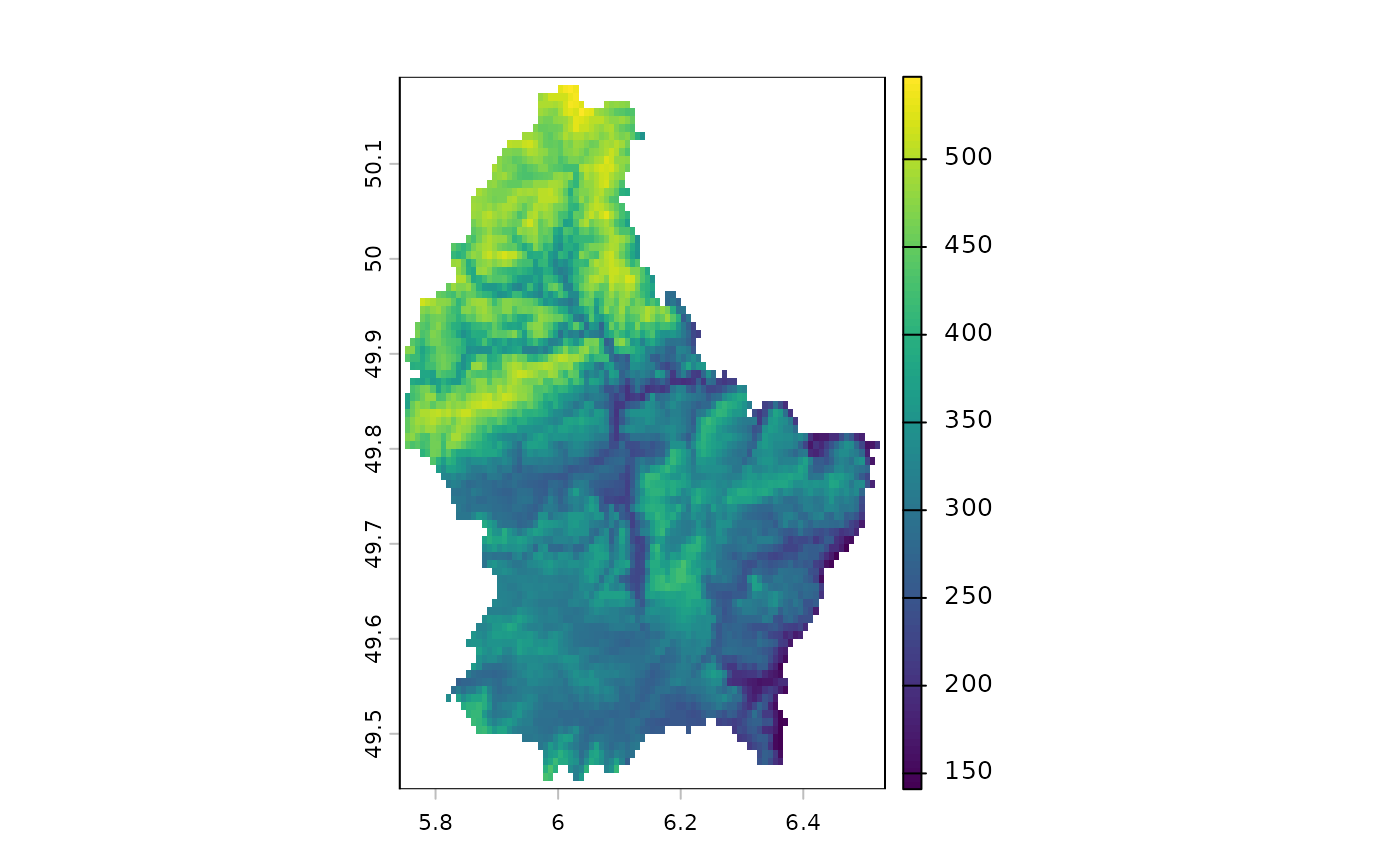plot(r, type="interval")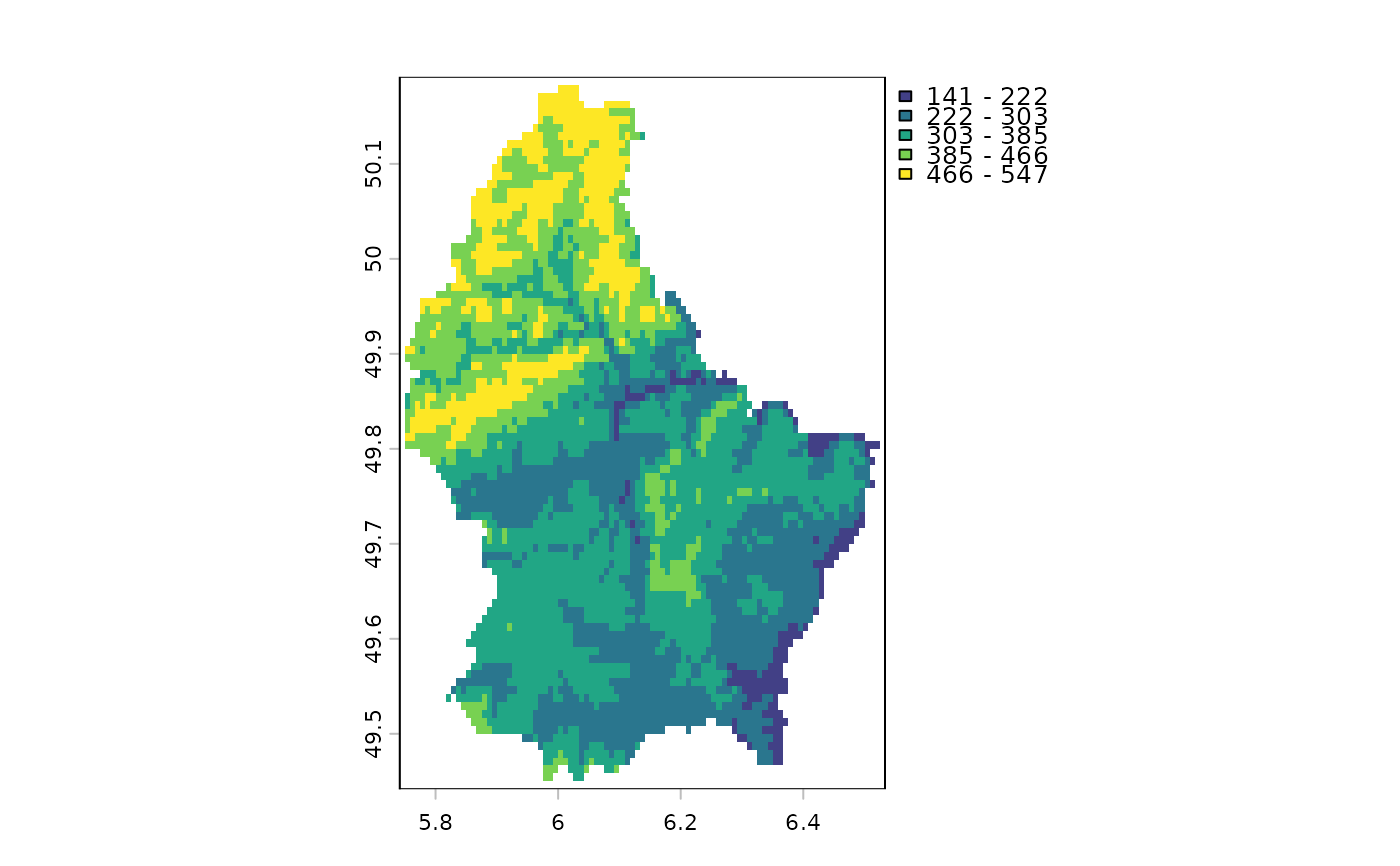e <- c(6.37, 6.41, 49.9, 50.1)
plot(r, plg=list(ext=e, title="Legend\nTitle", title.cex=0.9),
pax=list(side=1:4, retro=TRUE))
north(cbind(5.8, 50.1))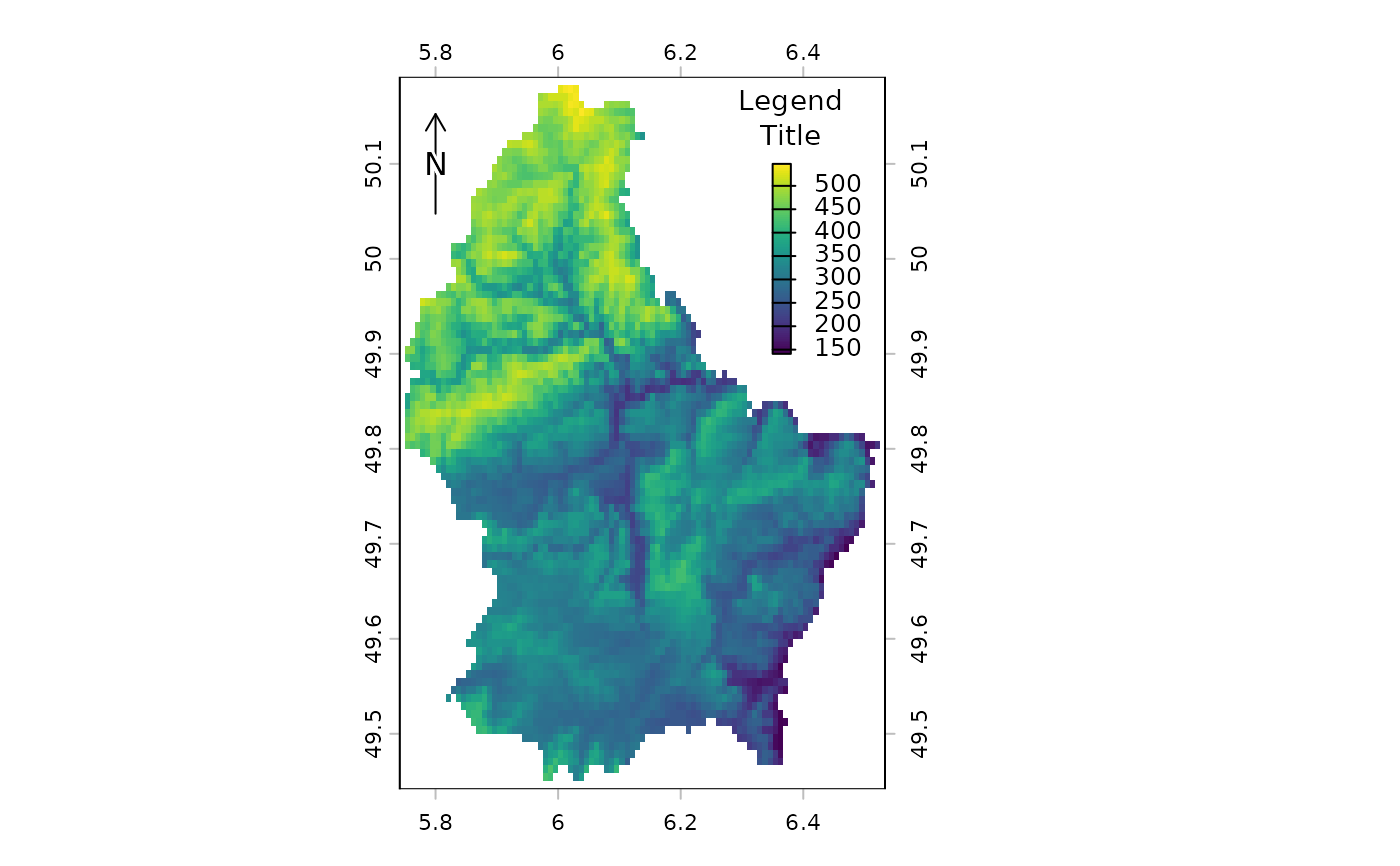d <- classify(r, c(100,200,300,400,500,600))
plot(d, type="classes")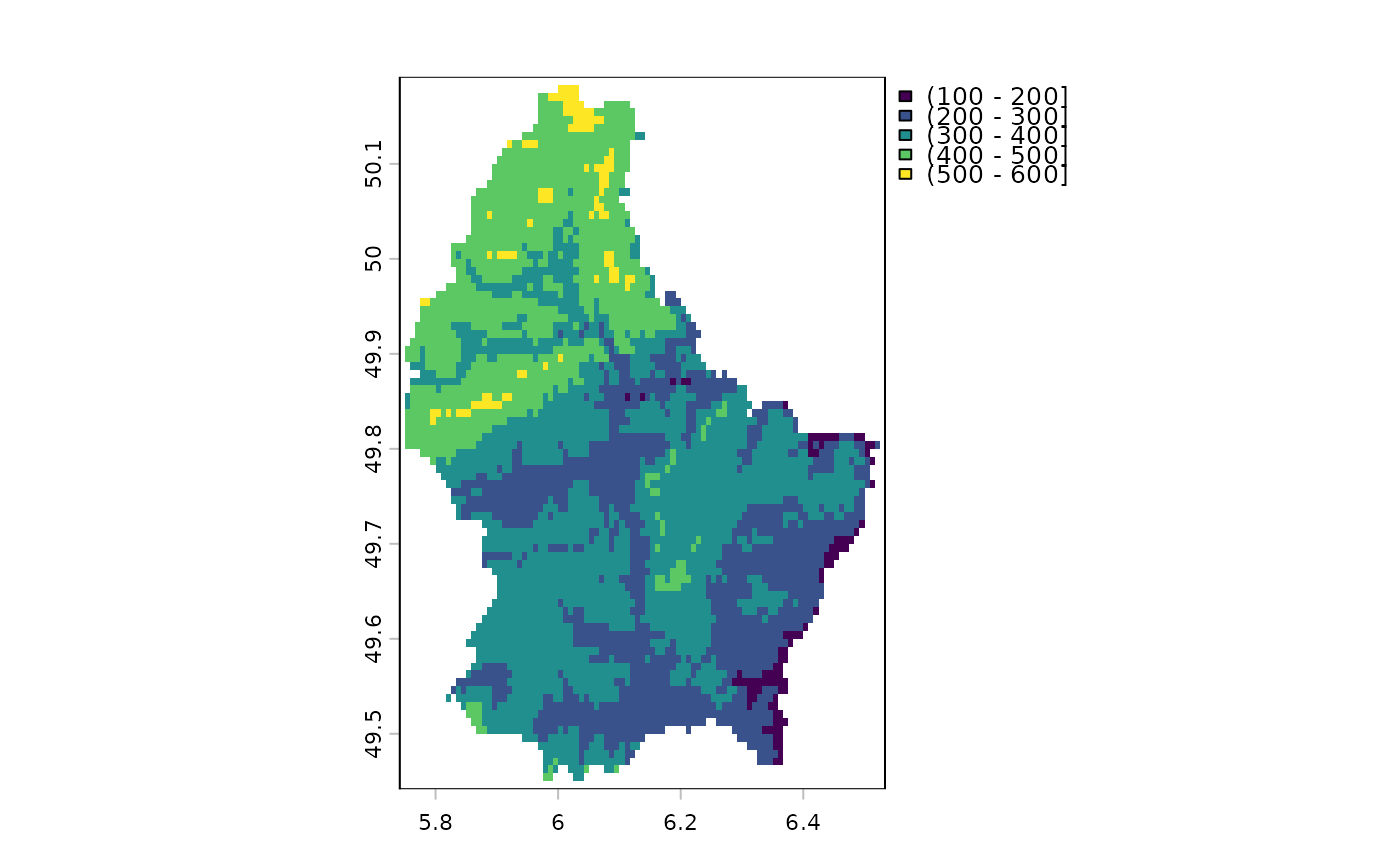plot(d, type="interval", breaks=1:5)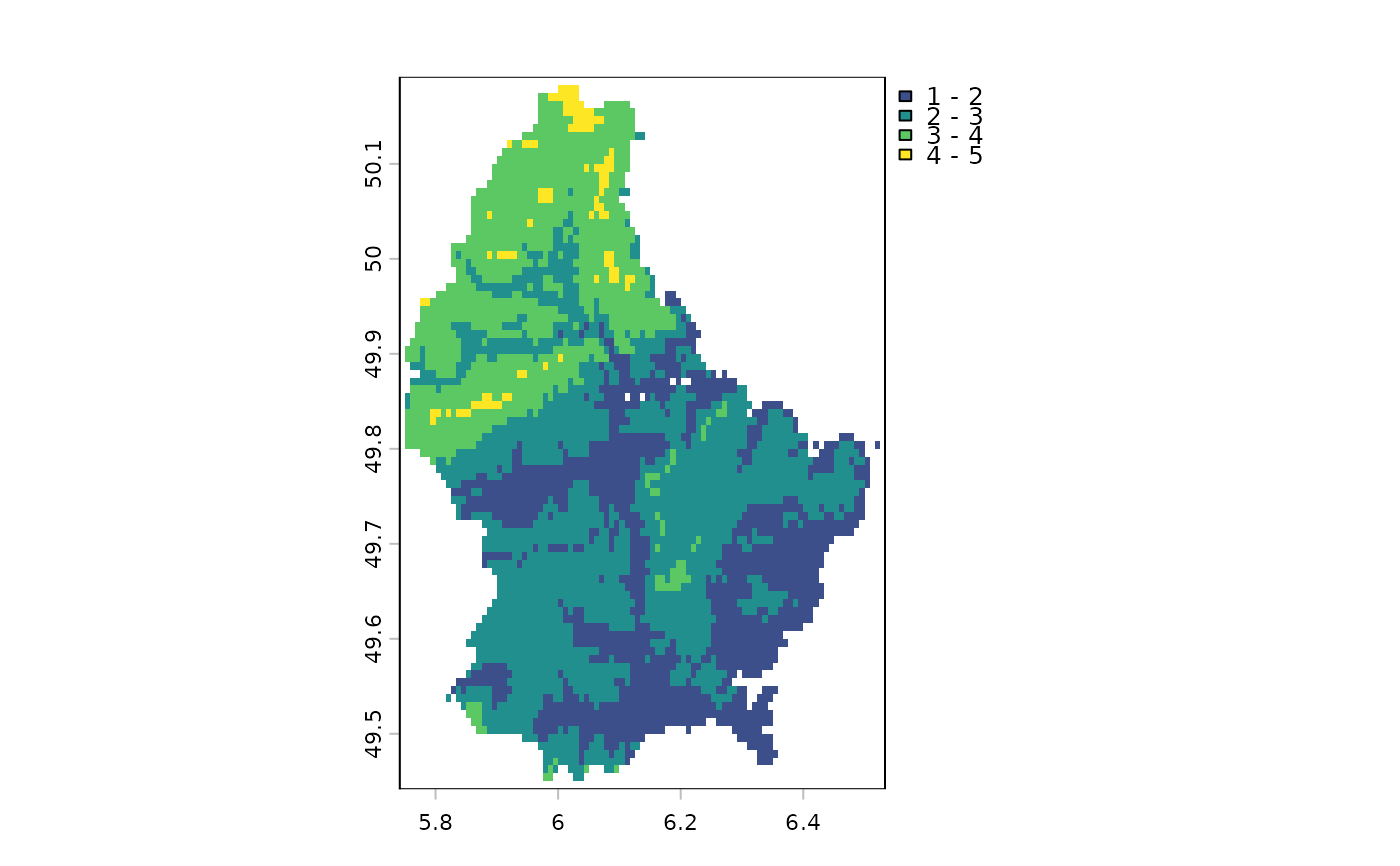plot(d, type="interval", breaks=c(1,4,5), plg=list(legend=c("1-4", "4-5")))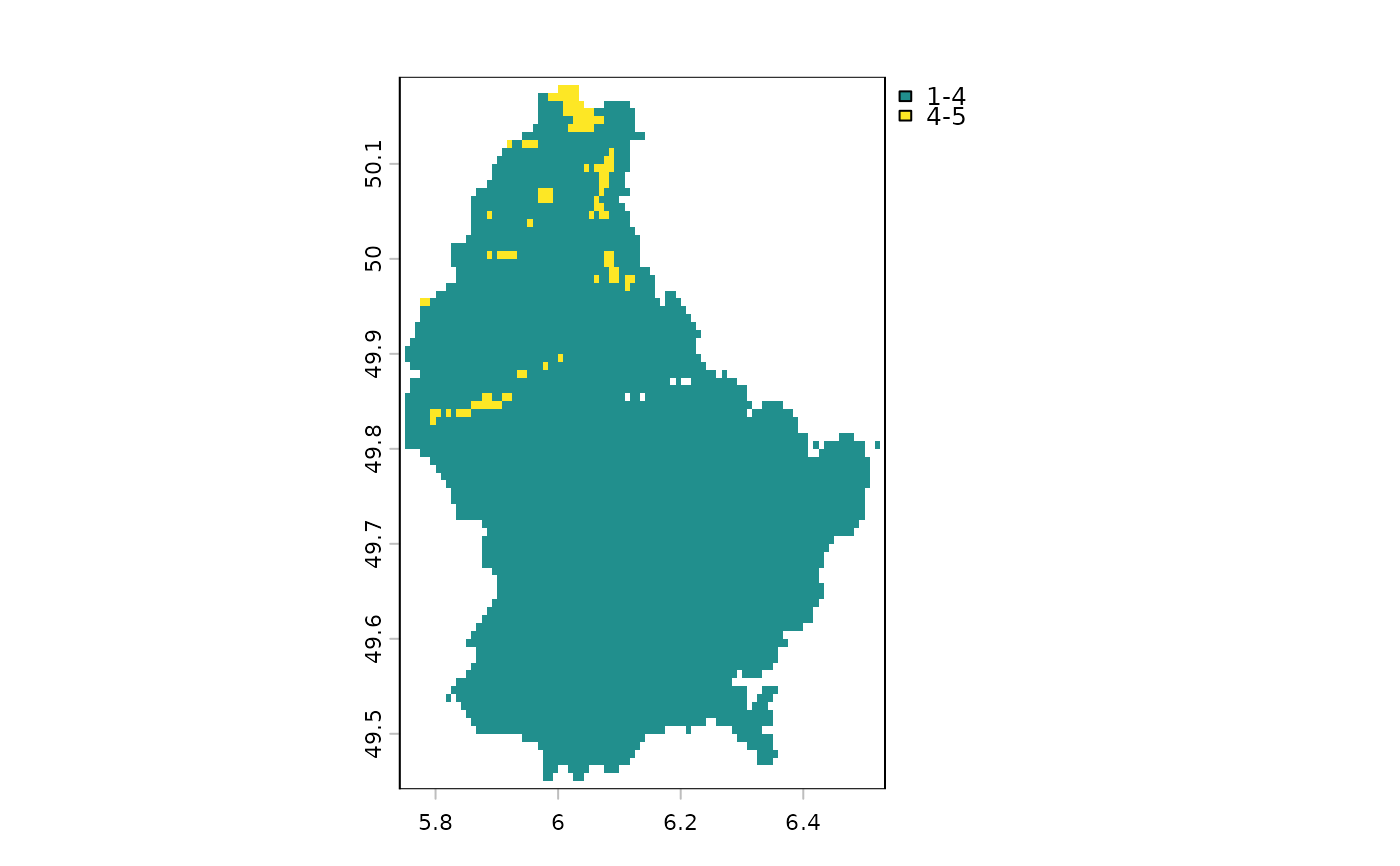plot(d, type="classes", xlim=c(5.6, 6.6),
plg=list(legend=c("Mr", "Xx", "As", "Zx", "Bb"), x="bottomleft"))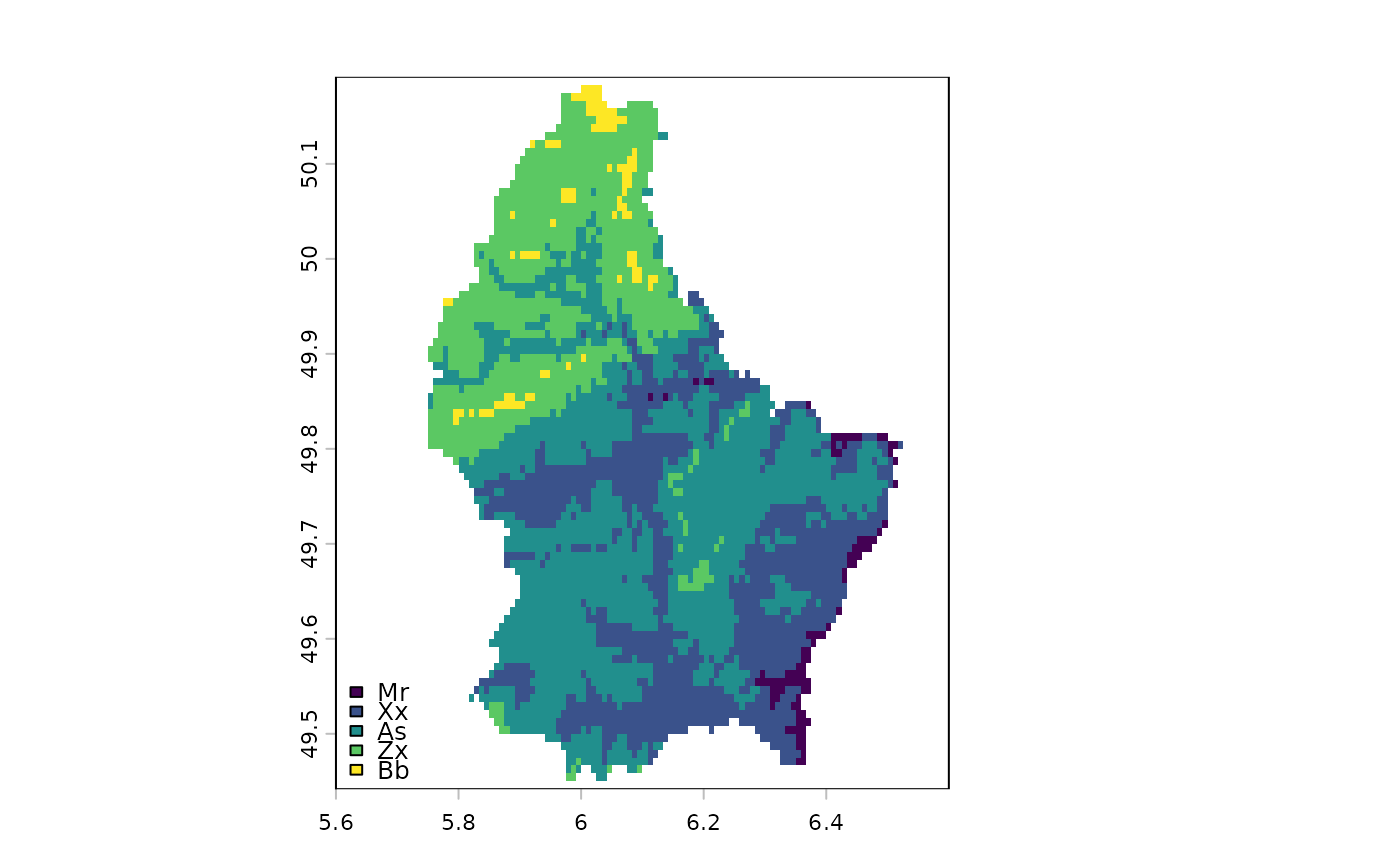x <- trunc(r/200)
levels(x) <- data.frame(id=0:2, element=c("earth", "wind", "fire"))
plot(x, plg=list(x="topright"),mar=c(2,2,2,2))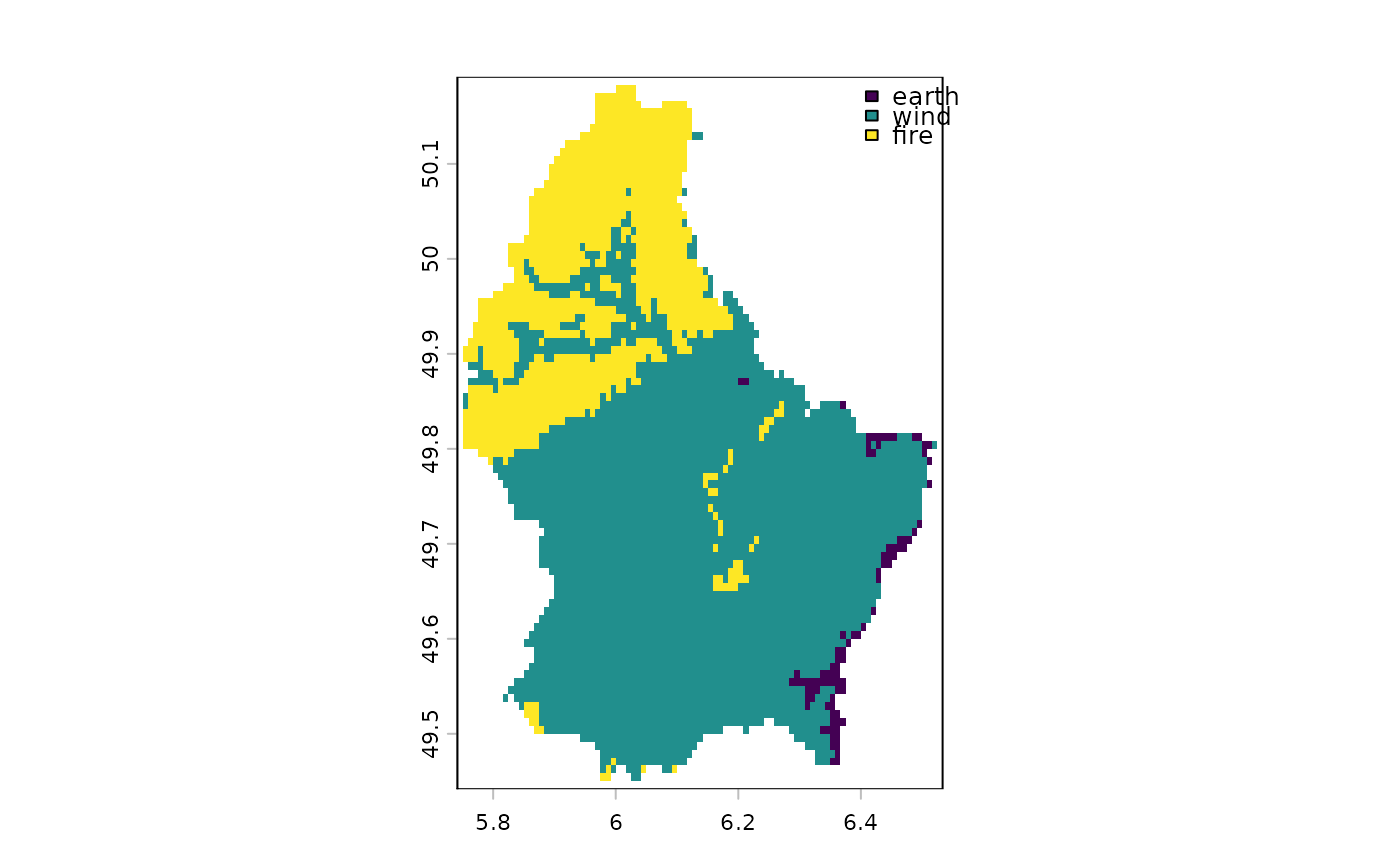# two plots with the same legend
dev.new(width=6, height=4, noRStudioGD = TRUE)
par(mfrow=c(1,2))
plot(r, range=c(50,600), mar=c(1,1,1,4))
plot(r/2, range=c(50,600), mar=c(1,1,1,4))

# as we only need one legend:
par(mfrow=c(1,2))
plot(r, range=c(50,600), mar=c(2, 2, 2, 2), plg=list(size=0.9, cex=.8),
pax=list(side=1:2, cex.axis=.6), box=FALSE)
#text(182500, 335000, "Two maps, one plot", xpd=NA)
plot(r/2, range=c(50,600), mar=c(2, 2, 2, 2), legend=FALSE,
pax=list(side=c(1,4), cex.axis=.6), box=FALSE)

par(oldpar)

# multi-layer with RGB
s <- rast(system.file("ex/logo.tif", package="terra"))
s
#> class       : SpatRaster
#> dimensions  : 77, 101, 3  (nrow, ncol, nlyr)
#> resolution  : 1, 1  (x, y)
#> extent      : 0, 101, 0, 77  (xmin, xmax, ymin, ymax)
#> coord. ref. : Cartesian (Meter)
#> source      : logo.tif
#> colors RGB  : 1, 2, 3
#> names       : red, green, blue
#> min values  :   0,     0,    0
#> max values  : 255,   255,  255
plot(s)
# remove RGB
plot(s*1)
# or use layers
plot(s, 1)
plot(s, 1:3)

# fix legend by linking values and colors

x = rast(nrows = 2, ncols = 2, vals=1)
y = rast(nrows = 2, ncols = 2, vals=c(1,2,2,1))
cols = data.frame(id=1:2, col=c("red", "blue"))
plot(c(x,y), col=cols)

r  = rast(nrows=10, ncols=10, vals=1:100)
dr = data.frame(from=c(5,33,66,150), to=c(33, 66, 95,200), col=rainbow(4))
plot(r, col=dr)

### SpatVector

f <- system.file("ex/lux.shp", package="terra")
v <- vect(f)

plot(v)

plot(v, "NAME_2", col=rainbow(12), border=c("gray", "blue"), lwd=3)

plot(v, 2, pax=list(side=1:2), plg=list(x=6.16, y=50.17, cex=.8), xlim=c(5.7, 6.7))

plot(v, 4, pax=list(side=1:2), plg=list(x=6.2, y=50.2, ncol=2), main="", box=FALSE)

plot(v, 1, plg=list(x=5.8, y=49.37, horiz=TRUE, cex=1.1), main="", mar=c(5,2,0.5,0.5))

plot(v, density=1:12, angle=seq(18, 360, 20), col=rainbow(12))

plot(v, "AREA", type="interval", breaks=3, mar=c(3.1, 3.1, 2.1, 3.1),
plg=list(x="topright"), main="")

plot(v, "AREA", type="interval", breaks=c(0,200,250,350),
mar=c(2,2,2,2), xlim=c(5.7, 6.75),
plg=list(legend=c("<200", "200-250", ">250"), cex=1, bty="o",
x=6.3, y=50.15, box.lwd=2, bg="light yellow", title="My legend"))